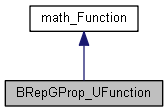# BRepGProp_UFunction Class Reference

This class represents the integrand function for computation of an inner integral. The returned value depends on the value type and the flag IsByPoint. More...

`#include <BRepGProp_UFunction.hxx>`

Inheritance diagram for BRepGProp_UFunction:[legend]

## Public Member Functions

BRepGProp_UFunction (const BRepGProp_Face &theSurface, const gp_Pnt &theVertex, const Standard_Boolean IsByPoint, const Standard_Address theCoeffs)
Constructor. Initializes the function with the face, the location point, the flag IsByPoint and the coefficients theCoeff that have different meaning depending on the value of IsByPoint. If IsByPoint is equal to Standard_True, the number of the coefficients is equal to 3 and they represent X, Y and Z coordinates (theCoeff, theCoeff and theCoeff correspondingly) of the shift, if the inertia is computed with respect to the point different then the location. If IsByPoint is equal to Standard_False, the number of the coefficients is 4 and they represent the combination of plane parameters and shift values. More...

void SetValueType (const GProp_ValueType theType)
Setting the type of the value to be returned. More...

void SetVParam (const Standard_Real theVParam)
Setting the V parameter that is constant during the integral computation. More...

virtual Standard_Boolean Value (const Standard_Real X, Standard_Real &F)
Returns a value of the function. More...Public Member Functions inherited from math_Function
virtual Standard_Integer GetStateNumber ()
returns the state of the function corresponding to the latest call of any methods associated with the function. This function is called by each of the algorithms described later which defined the function Integer Algorithm::StateNumber(). The algorithm has the responsibility to call this function when it has found a solution (i.e. a root or a minimum) and has to maintain the association between the solution found and this StateNumber. Byu default, this method returns 0 (which means for the algorithm: no state has been saved). It is the responsibility of the programmer to decide if he needs to save the current state of the function and to return an Integer that allows retrieval of the state. More...

## Detailed Description

This class represents the integrand function for computation of an inner integral. The returned value depends on the value type and the flag IsByPoint.

The type of returned value is the one of the following values:

• GProp_Mass - volume computation.
• GProp_CenterMassX, GProp_CenterMassY, GProp_CenterMassZ - X, Y and Z coordinates of center of mass computation.
• GProp_InertiaXX, GProp_InertiaYY, GProp_InertiaZZ, GProp_InertiaXY, GProp_InertiaXZ, GProp_InertiaYZ
• moments of inertia computation.

If the flag IsByPoint is set to Standard_True, the value is returned for the region of space that is delimited by a surface and a point. Otherwise all computations are performed for the region of space delimited by a surface and a plane.

## Constructor & Destructor Documentation

 BRepGProp_UFunction::BRepGProp_UFunction ( const BRepGProp_Face & theSurface, const gp_Pnt & theVertex, const Standard_Boolean IsByPoint, const Standard_Address theCoeffs )

Constructor. Initializes the function with the face, the location point, the flag IsByPoint and the coefficients theCoeff that have different meaning depending on the value of IsByPoint. If IsByPoint is equal to Standard_True, the number of the coefficients is equal to 3 and they represent X, Y and Z coordinates (theCoeff, theCoeff and theCoeff correspondingly) of the shift, if the inertia is computed with respect to the point different then the location. If IsByPoint is equal to Standard_False, the number of the coefficients is 4 and they represent the combination of plane parameters and shift values.

## Member Function Documentation

 void BRepGProp_UFunction::SetValueType ( const GProp_ValueType theType )

Setting the type of the value to be returned.

 void BRepGProp_UFunction::SetVParam ( const Standard_Real theVParam )

Setting the V parameter that is constant during the integral computation.

 virtual Standard_Boolean BRepGProp_UFunction::Value ( const Standard_Real X, Standard_Real & F )
virtual

Returns a value of the function.

Implements math_Function.

The documentation for this class was generated from the following file: